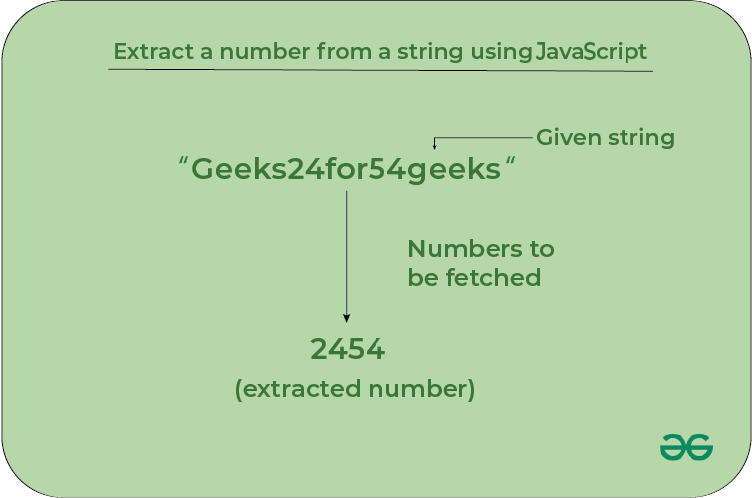# Extract a number from a string using JavaScript

In this article, we will learn how we can extract the numbers if exist in a given string. we will have a string and we need to print the numbers that are present in the given string in the console.These are the following methods by using these we can solve the problems:

## Method 1: Using the JavaScript match method with regEx

The number from a string in javascript can be extracted into an array of numbers by using the match method. This function takes a regular expression as an argument and extracts the number from the string. The regular expression for extracting a number is (/(\d+)/).

Example 1: This example uses the match() function to extract numbers from strings with regular expressions.

## Javascript

 `// Function to extract numbers` `function` `myGeeks() {`   `    ``// Input string` `    ``let str = ``"jhkj7682834"``;` `    ``console.log(str)`   `    ``// Using match with regEx` `    ``let matches = str.match(/(\d+)/);` `    `  `    ``// Display output if number extracted` `    ``if` `(matches) {` `        ``console.log(matches);` `    ``}` `}`   `// Function call` `myGeeks();`

Output

```jhkj7682834
7682834
```

Example 2: This example uses the match() function to extract numbers from strings.

## Javascript

 `// Function to extract numbers` `function` `myGeeks() {`   `    ``// Input string` `    ``let str = ``"foo35bar5jhkj88"``;` `    ``console.log(str)`   `    ``// Using match with regEx` `    ``let matches = str.match(/\d+/g);` `    `  `    ``// Display output if number extracted` `    ``if` `(matches) {` `        ``console.log(matches +``', '``+ matches+``', '` `+ matches);` `    ``}` `}`   `// Function call` `myGeeks();`

Output

```foo35bar5jhkj88
35, 5, 88
```

## Method 2: Using the JavaScript reduce() method with the spread operator

The Javascript arr.reduce() method in JavaScript is used to reduce the array to a single value and executes a provided function for each value of the array (from left to right) and the return value of the function is stored in an accumulator.

Example: This example spread operator to convert into an array and the reduce method to extract all the numbers from the string.

## Javascript

 `// Function to extract numbers` `function` `myGeeks() {` `    ``// Input string` `    ``let str = ``"jhkj7682834"``;` `    ``let c = ``"0123456789"``;` `    ``console.log(str);` `    ``function` `check(x) {` `        ``return` `c.includes(x) ? ``true` `: ``false``;` `    ``}` `    ``let matches = [...str].reduce(` `        ``(x, y) => (check(y) ? x + y : x),``""``);`   `    ``// Display output if number extracted` `    ``if` `(matches) {` `        ``console.log(matches);` `    ``}` `}`   `// Function call` `myGeeks();`

Output

```jhkj7682834
7682834
```

## Method 3: Using the JavaScript replace() method with regEx

The string.replace() is an inbuilt method in JavaScript that is used to replace a part of the given string with another string or a regular expression. The original string will remain unchanged.

Example: In this example, we will use the JavaScript replace() method with regEx to extract the numbers out of given string

## Javascript

 `// Function to extract numbers` `function` `myGeeks() {` `    ``// Input string` `    ``let str = ``"jhkj7682834"``;` `    ``console.log(str);`   `    ``// Using match with regEx` `    ``let matches = str.replace(/[^0-9]/g, ``""``);`   `    ``// Display output if number extracted` `    ``if` `(matches) {` `        ``console.log(matches);` `    ``}` `}`   `// Function call` `myGeeks();`

Output

```jhkj7682834
7682834
```

JavaScript is best known for web page development but it is also used in a variety of non-browser environments. You can learn JavaScript from the ground up by following this JavaScript Tutorial and JavaScript Examples.

Whether you're preparing for your first job interview or aiming to upskill in this ever-evolving tech landscape, GeeksforGeeks Courses are your key to success. We provide top-quality content at affordable prices, all geared towards accelerating your growth in a time-bound manner. Join the millions we've already empowered, and we're here to do the same for you. Don't miss out - check it out now!

Previous
Next